# Transimpedance amplifier designs for high-performance, cost-sensitive smoke detector applications

This post is co-authored by Collin Wells.

Photodiode-based light sensing is a common technique where operational amplifiers (op amps) are used to condition a photodiode sensor for a wide range of applications. An example of this is in smoke detectors, where a photoelectric smoke alarm is used to identify the presence of smoke in the chamber.

A photodiode sensor produces a current proportional to the light level presented to it. Depending on the application, the photodiode is operated in either a photovoltaic or photoconductive mode; each has its own merits, which Bruce Trump discussed in detail in this post from his blog, The Signal.

In a smoke detector system, the photodiode operates in a photoconductive mode, meaning you will typically use a transimpedance amplifier to amplify the photodiode current. In photoconductive mode, the photodiode is held at a zero-volt (Figure 1a) or reverse voltage bias (Figure 1b), preventing it from forward biasing.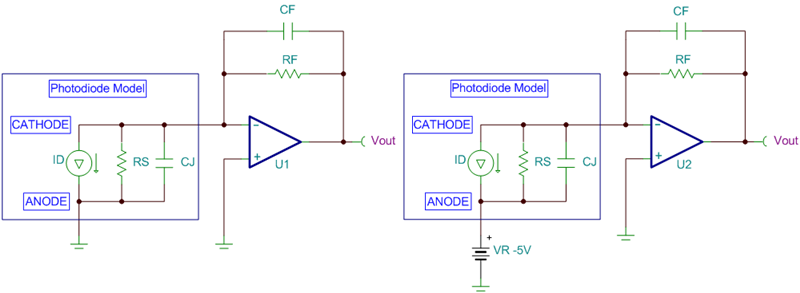Figure 1: Photodiode in photoconductive mode with zero-volt bias (a); or reverse-voltage bias (b)

Equation 1 calculates the direct current (DC) transfer function for the circuits shown in Figure 1 (note that the photodiode current (iD) is flowing away from the op-amp inverting node):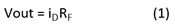The three-step process outlined in John Caldwell’s series on transimpedance amplifiers (see part 3, “What op amp bandwidth do I need?”) determines the minimum required op-amp gain bandwidth for transimpedance configurations. The minimum bandwidth is based on the required transimpedance gain and signal bandwidth, along with the total capacitance presented to the inverting node of the op amp. The diode capacitance often dominates the inverting-node capacitance, but don’t forget to include the effects of the op-amp input capacitance. We summarized the three steps explained in John’s posts here for quick reference.

1. Choose the maximum feedback capacitance (CF) based on the feedback resistor (RF) and the signal -3dB bandwidth (fP) (Equation 2):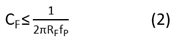2. Calculate the total capacitance (CIN) at the inverting input of the amplifier. For the circuits shown in Figure 1, this is equal to Equation 3: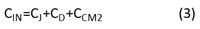where CJ is the diode junction capacitance, CD is the op-amp differential input capacitance and CCM2 is the op-amp inverting input common-mode input capacitance.

3. Calculate the minimum required op-amp gain bandwidth product (fGBW) (Equation 4):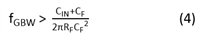By following these three simple steps, you can avoid many of the stability and performance issues commonly associated with transimpedance amplifier circuits by selecting an amplifier with sufficient bandwidth to perform the required transimpedance gain at the desired signal bandwidth. Op amps with GBWs between 1 - 20MHz are well suited for smoke detector applications because they are able to amplify the low-level signals in the system to sufficient levels while maintaining stability.

Along with meeting bandwidth requirements, the op amp must also meet the system’s DC accuracy requirements. The most important DC specification in many transimpedance applications is the input bias current (iB) of the op amp. iB will directly sum or subtract with the input signal current, which can cause large errors depending on the magnitude of iB compared to the signal current. In smoke detector applications, this DC voltage enables system designers to set the thresholds for the amount of smoke detected before an action is taken in the system.

The example shown in Figure 2 uses a 5MΩ resistor to apply a 5MV/A gain to a 100nA full-scale input current. With the input bias current set to 0A, the full-scale output voltage is 500mV – which is expected based on the transfer function in Equation 1. The circuit on the right in Figure 2 displays the effects of the same circuit with an op amp iB of 10nA. In this case, the output voltage is 450mV, which shows that the 10nA input bias current caused a 50mV (or 10%) error from the ideal 500mV output signal.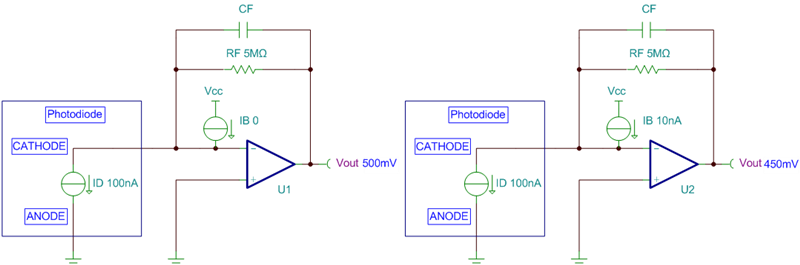Figure 2: Input bias current effects in transimpedance amplifier circuits

Equation 5 calculates the percentage error of the full-scale range (%FSR) based on the full-scale input current (iIN_FS) and the op amp’s iB: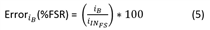The TLV6001 device is part of a family of high-performance general-purpose amplifiers for a wide variety of cost-conscious transimpedance applications, such a smoke detectors. This is due to the op amps balance between GBW (1 MHz), low Iq (100 µA), low input bias current (1 pA) and low input capacitance (6pF). Other key features that make this family attractive for system designers in smoke detectors are the RRIO swings and the EMI hardened inputs that help reject interference from extrinsic noise sources.

Table 1 lists different transimpedance gain and bandwidth combinations for the TLV6001 based on Equations 1 through 4. Be sure to keep the total input capacitance below the maximum input capacitance (CIN­_MAX) to avoid stability issues.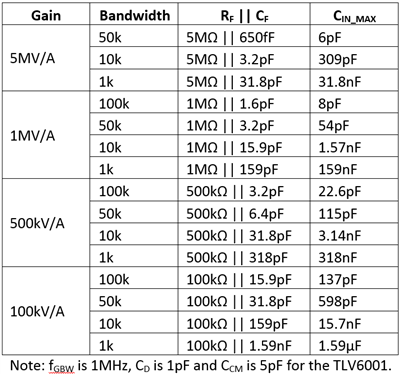Table 1: Quick design calculator for TLV6001 transimpedance applications

Figure 3 shows the simulated step-response results for a 1MV/A gain and 50kHz bandwidth with the maximum 54pF of input capacitance from the photodiode. The output overshoot and ringing are minimal, indicating a stable design.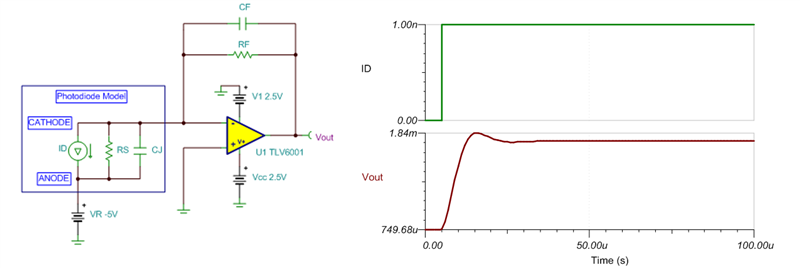Figure 3: TLV6001 step-response results; gain = 1MV/A, bandwidth = 50kHz

Smoke detector applications use op amps in the transimpedance configuration to amplify low-level photodiode currents. Designing the transimpedance circuit for smoke detectors can be simplified to a few easy steps. First, follow the three steps from John’s blog posts to select the required op-amp bandwidth. Then sort the remaining results to find a device with an iB specification that meets the system’s DC requirements. The TLV600x devices highlighted in this blog are a great family of products to design with for cost-sensitive transimpedance applications.